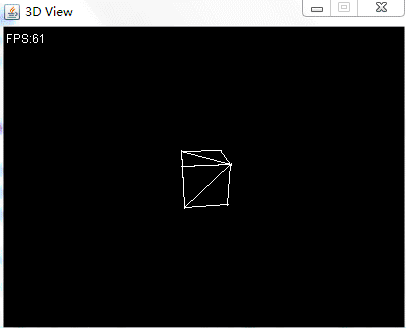## 目标

• 将三角网格的背面（看不见的部分）剔除掉。

## 实现

### 变换到观察空间

``````/**
* 渲染3D场景
*
* @param imageRaster
* @param camera
*/
public void render(ImageRaster imageRaster, Camera camera) {
// 世界变换矩阵
Matrix4f worldMat = transform.toTransformMatrix();
// 观察-投影变换矩阵...

// 模型-观察变换矩阵
Matrix4f mv = camera.getViewMatrix().mult(worldMat);

// 模型-观察-投影变换矩阵...
// 视口变换矩阵...
// 用于保存变换后的向量坐标...

// 遍历所有三角形
for (int i = 0; i < indexes.length - 2; i += 3) {
int a = indexes[i];
int b = indexes[i + 1];
int c = indexes[i + 2];

Vector3f va = positions[a];
Vector3f vb = positions[b];
Vector3f vc = positions[c];

// 在摄像机空间进行背面消隐
if (cullBackFace(mv.mult(va), mv.mult(vb), mv.mult(vc)))
continue;

// 使用齐次坐标计算顶点...
// 模型-观察-透视 变换...
// 透视除法...
// 把顶点位置修正到屏幕空间...
// 画三角形...
}
}
``````

### 判断是否为背面

A、B、C三个向量是按照顶点索引的顺序传入的，根据这三个向量，就可以计算出表面法线的方向。

``````faceNormal = (B-A) × (C-B)
``````

``````faceNormal dot C
``````

``````/**
* 剔除背面
*
* @param a
* @param b
* @param c
* @return
*/
protected boolean cullBackFace(Vector3f a, Vector3f b, Vector3f c) {

// 计算ab向量
Vector3f ab = b.subtract(a, a);

// 计算bc向量
Vector3f bc = c.subtract(b, b);

// 计算表面法线
Vector3f faceNormal = ab.crossLocal(bc);

return faceNormal.dot(c) >= 0;
}
``````

### 测试用例